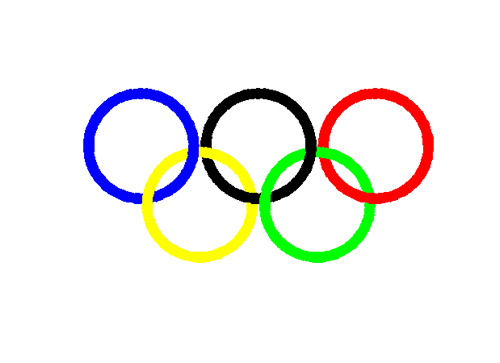Olympic fever

Watching the Olympics opening ceremonies, I’m reminded of my very first MATLAB program. I was a beleaguered freshman in a scientific computation class for which I did not meet the prerequisites. The first problem set was a warm-up to get used to doing assignments using MATLAB-files. To test our plot skills, the professor had us plot Olympic rings in a figure window.

It was an interesting challenge – learning trig functions, the subtleties of plot, and the twist of interlocking rings of different colors. Unfortunately, I did not start archiving my assignments until my sophomore year, so I don’t have that original M-file to share. Here is an unelegant, non-vectorized replica that I came up with today. I’m sure Loren could come up with something more clever, but it is a fun thing to do and in the spirit of the times.%rings.m plots olympic rings
N = 100;
angle = linspace(pi/4,9*pi/4,N); %all the way around

% Make the x and y's for each of the five circles
xb = cos(angle) * 0.9;
yb = sin(angle) * 0.9;

xy = cos(angle) * 0.9 + 1;
yy = sin(angle) * 0.9 - 1;

xk = cos(angle) * 0.9 + 2;
yk = sin(angle) * 0.9;

xg = cos(angle) * 0.9 + 3;
yg = sin(angle) * 0.9 - 1;

xr = cos(angle) * 0.9 + 4;
yr = sin(angle) * 0.9;

% Make the Figure
figure
hold on
plot(xb(1:3*N/4),yb(1:3*N/4),'b','linewidth',10);
plot(xy(N/4:N),yy(N/4:N),'y','linewidth',10)

plot(xk(1:3*N/4),yk(1:3*N/4),'k','linewidth',10);
plot(xy(1:N/4),yy(1:N/4),'y','linewidth',10);
plot(xb(3*N/4:end),yb(3*N/4:end),'b','linewidth',10);

plot(xr(1:N/2),yr(1:N/2),'r','linewidth',10);
plot(xg(1:N),yg(1:N),'g','linewidth',10);

plot(xk(3*N/4:N),yk(3*N/4:N),'k','linewidth',10);
plot(xr(N/2:N),yr(N/2:N),'r','linewidth',10);

%make the axis pretty
axis equal
axis off
set(gca,'XLim',[-1.2 5.2])
set(gcf,'Color',[1 1 1])

What does this have to do with the desktop? Well, not much. I thought I’d come up with some gold-medal metaphor, but nothing uncontrived comes to mind. Instead I’ll leave you with a request for MATLAB programs analyzing olympic results, or even better, predicting olympic results. To get started, I recommend using urlread with an updated results site.

|